Home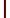HockeyBuzzRegisterLogin
"The egg came before the chicken, so you must listen"
Hamilton, ON • Canada • 22 Years Old • Male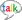None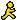eggshmeg35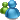[email protected]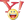[email protected]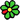None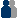Add to Friends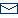Send Message
Some of you may have followed my predictions for the first two rounds of this year's NHL entry draft. Here are the results of my predictions comparing them to where they actually ended up. They are ordered in how I picked them, the number on the right is where they actually ended up, and the third number is how far off I was:

1. P. Kane - 1 = 0
2. K. Turris - 3 = +1
3. A. Cherepanov - 17 = +14
4. S. Gagner - 6 = +2
5. K. Alzner - 5 = 0
6. J. Vanriemsdyk - 2 = -4
7. J. Voracek - 7 = 0
8. A. Esposito - 20 = +12
9. R. McDonagh - 12 = +3
10. K. Ellerby - 10 = 0
11. Z. Hamill - 8 = -3
12. K. Veilleux - 51 = +39
13. L. Couture - 9 = -4
14. N. Petrecki - 28 = +14
15. L. Eller - 13 = -2
16. M. Pacioretty - 22 = +6
17. M. Mayorov - 94 = +77
18. D. Tyrell - 47 = +29
19. C. Gilles - 16 = -3
20. M. Hoeffel - 57 = +37
21. J. Andersson - 88 = +67
22. M. Goncharov - 123 = + 101
23. J. Blum - 23 = 0
24. D. Perron - 26 = +2
25. B. Sutter - 11 = -14
26. K. Shattenkirk - 14 = -12
27. C. Cohen - 45 = +18
28. O. Moller - 52 = +24
29. M. Backlund - 24 = -5
30. T. Cross - 35 = +5
31. T. Hickey - 4 = -27
32. S. Legein - 37 = +5
33. M. Repik - 40 = +7
34. J. Smith - 54 = +20
35. L. Cunti - 75 = +40
36. B. Sweatt - 38 = +2
37. T. Ellington - 33 = -4
38. B. Maclean - 32 = -6
39. M. Gratchev - 106 = +77
40. N. Spaling - 58 = +18
41. P. White - 25 = -16
42. J. O'Brien - 29 = -13
43. L. Macmillan - 19 = -24
44. R. Bashkirov - 60 = +16
45. T. Galiardi - 55 = +10
46. T. Brennan - 31 = -15
47. A. Aliu - 56 = +9
48. B. Ryan - 114 = +66
49. J. Gistedt - 36 = -13
50. B. Cameron - 82 = +32
51. K. Patterson - 113 = +62
52. M. Tanguay - 69 = +17
53. T. Ruth - 46 = -7
54. N. Lucenius - 115 = +61
55. R. Bortuzzo - 78 = +23
56. M. Katic - 62 = +6
57. A. Plante - 15 = -42
58. N. Palmieri - 79 = +21
59. K. Marshall - 41 = -18
60. D. Bowman - 72 = +12
61. E. Doyle - undrafted = ??

I think I did fairly well. I stayed relatively close with some of the later picks, so I am happy. Not one of my better predicitons, but I'll take it. I ended up getting 5 players perfect and only 7 players more than 50 spots off.
Filed Under:   prospects   draft   entry   nhl   eggshmeg
March 8, 2022 2:09 AM ET | Delete
The reason for me wanting to plunk down some greenbacks and take a trip in the Wayback Machine. concrete driveway